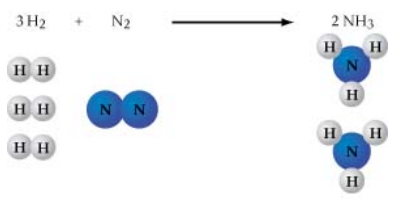Problem: Now that we have put coefficients of 3 in front of CaSO4 and CaCl2, what coefficient should go in front of AlCl3 to balance both the Cl atoms and the Al atoms?3 CaSO4 + ?AlCl3 → 3 CaCl2 + Al2(SO4)3Express your answer numerically as an integer.According to the law of conservation of mass, matter cannot be created or destroyed. Therefore, a chemical equation must show the same number of each kind of atom in the reactants as it does in the products. As shown in the figure, the balanced equation 3 H2 + N2 → 2 NH3 has 6 atoms of hydrogen and 2 atoms of nitrogen on each side of the arrow. One is often presented with unbalanced chemical equations for which one must supply the coefficients.The following equation is not balanced:CaSO4 + AlCl3 → CaCl2 + Al2(SO4)3

FREE Expert Solution
92% (404 ratings)Problem Details

Now that we have put coefficients of 3 in front of CaSO4 and CaCl2, what coefficient should go in front of AlCl3 to balance both the Cl atoms and the Al atoms?

3 CaSO4 + ?AlCl3 → 3 CaCl2 + Al2(SO4)3

According to the law of conservation of mass, matter cannot be created or destroyed. Therefore, a chemical equation must show the same number of each kind of atom in the reactants as it does in the products. As shown in the figure, the balanced equation 3 H2 + N2 → 2 NH3 has 6 atoms of hydrogen and 2 atoms of nitrogen on each side of the arrow. One is often presented with unbalanced chemical equations for which one must supply the coefficients.The following equation is not balanced:

CaSO4 + AlCl3 → CaCl2 + Al2(SO4)3